• 提出一种基于模糊神经网络进行数据挖掘的新方法。构成模糊神经网络的模糊化层采用高斯函数计算5个模糊隶属度，高斯函数需要的均值、方差以及隶属度的中心值都可通过预先计算采集到的数据得到。模糊推理层采用取小取...
• 1.人工神经网络： BP神经网络 误差逆传播算法训练的多层前馈网络 LM神经网络 基于梯度下降和牛顿结合点多层前馈网络，迭代少，精度高 RBF径向积神经网络 可以任意精度逼近连续函数，适合解决...
1.人工神经网络：BP神经网络
误差逆传播算法训练的多层前馈网络
LM神经网络
基于梯度下降和牛顿法结合点多层前馈网络，迭代少，精度高
RBF径向积神经网络
可以任意精度逼近连续函数，适合解决分类问题
FNN模糊神经网络
具有模糊系数或输入信号是模糊量的神经网络
GMDH神经网络
多项式神经网络，前馈神经网络中用于预测的不稳定结构网络
ANFIS自适应神经网络
全模糊结构，可自动产生、修正并高度概括规则
（训练数据需要TensorFlow、keras、pandas等相关64位Python库）
数据举例：

num

wealth

weekend

p_sale

num_sale

1

bad

yes

yes

high

2

bad

yes

yes

high

3

bad

yes

yes

high

4

bad

no

yes

high

5

bad

yes

yes

high

6

bad

no

yes

high

7

bad

yes

no

high

8

good

yes

yes

high

9

good

yes

no

high

。。。
data[data == u'yes'] = 1
data[data == u'high'] = 1
data[data != 1] = 0
x = data.iloc[:,:3].as_matrix().astype(int)
y = data.iloc[:,3].as_matrix().astype(int)

from keras.models import Sequential
from keras.layers.core import Dense, Activation

model = Sequential() #建立模型
model.add(Dense(input_dim = 3, output_dim = 10))
model.add(Activation('relu')) #用relu函数作为激活函数，能够大幅提供准确度
model.add(Dense(input_dim = 10, output_dim = 1))
model.add(Activation('sigmoid')) #由于是0-1输出，用sigmoid函数作为激活函数

model.compile(loss = 'binary_crossentropy', optimizer = 'adam')
#编译模型。由于我们做的是二元分类，所以我们指定损失函数为binary_crossentropy,求解方法我们指定用adam，还有sgd、rmsprop等可选

model.fit(x, y, nb_epoch = 500, batch_size = 10) #训练模型，学习500次
yp = model.predict_classes(x).reshape(len(y)) #分类预测

#from cm_plot import * #导入自行编写的混淆矩阵可视化函数
#cm_plot(y,yp).show() #显示混淆矩阵可视化结果
print y
print yp
展开全文python 机器学习 TensorFlow 算法
• 通过介绍评定模型，随机梯度下降，生物启发和感知器系统，讲师用视图和数学解析式详细地讲解了神经网络的运行过程以及原理. 课程大纲： 1、Stochastic Gradient Descent ( 随机梯度下降 ) 2、Neural ...
转载自：http://blog.csdn.net/feitianhu213/article/details/39899463

课程简介：

本节课主要介绍人工神经网络.通过介绍评定模型，随机梯度下降法，生物启发和感知器系统，讲师用视图和数学解析式详细地讲解了神经网络的运行过程以及原理.
课程大纲：

1、Stochastic Gradient Descent ( 随机梯度下降法 )

2、Neural Network Model ( 神经网络模型 )

3、Backpropagation Algorithm ( 反向传播算法 )

4、Summarize
1、Stochastic Gradient Descent

在上一节课里，我们利用梯度下降法找到最优的方向，然后向该方向移动一小步，但是很有可能会导致陷入一个差的局部最优解，而且每次移动都要计算全部的点，因此计算量大。那如果每次移动只看一个点，根据当前的点找到最优的方向进行移动，当所有的点都被选择后，理论上总的移动方向是跟之前的方法一致的，因为数学期望是相等的。但是利用随机的选取一个点，却有可能让我们逃脱非常差的局部最优点的陷阱，当然无法逃脱全部的陷阱。那是因为当你随便选取一点的时候，它的方向并不一定就是最优化的那个方向，因此下一步有可能移动到高点去，然后在新的位置重新寻找出路，这样就有更大的可能性获得较好的局部最优点。考虑下面的图片，在左图中，当我们第一次选择的初始化位置是第一个黑点的时候，那么根据整体梯度下降法，就会陷入那个非常糟糕的局部嘴有点去了。然而如果我们选择的是随机梯度下降法，就有可能因为某个点的方向是偏离最优点的而使得前进的方向是想着右边的，这样就可以跳过该陷阱了。由图中，根据整体梯度下降法，当遇到平缓区域的时候，该方法就会认为找到最优点了，就会停滞不前。而随机梯度下降法却由于不同点的方向不一致从而推动了算法继续向前移动，于是我们再次逃脱了该陷阱了。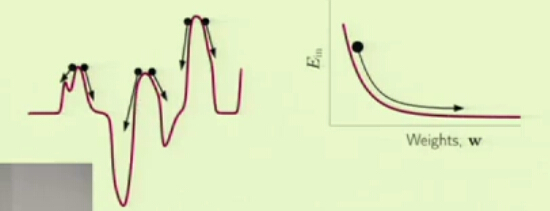应用随机梯度下降法有如下好处：

1、计算代价小，因为每次只选取一个点进行计算

2、随机性有助于逃脱局部最小点，找到较好的最小点

3、简单，因为每次只选取一个点进行计算
2、Neural Network Model ( 神经网络模型 )

在学习感知器模型的时候我们知道当输入数据点超过三个的时候，最坏情况下我们没办法把所有的点全部正确分类，即 dVC = 3。然而如果利用两个感知器模型进行分类，然后再综合这两个模型的结果得到最总的结果，我们就有办法把所有的 4 个点全部分类，如下图：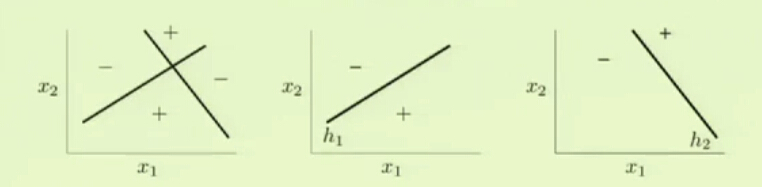对于更多的点，我们可以利用更多的模型进行分类。通过综合不同模型的结果，就有可能通过感知器模型找到最优的解（权重）。

利用以下图形进行直观的解释：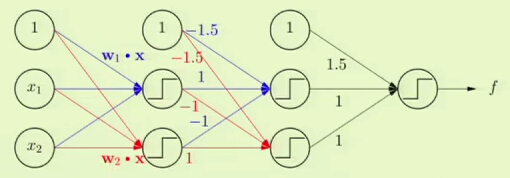上图中共有第一列是输入，后面三列表示三层模型，共 5 个感知器模型（每个圆圈表示一个感知器模型，也叫一个神经元或节点）。最后的感知器输出的就是最终的结果。

每一条线上都有一些数值，这些便是我们需要学习的权重（参数），开始的时候是未知的。上述模型其实就是一个神经网络。

理论上通过这些感知器的排列组合(任意个)，可以产生任何输出。

定义：

神经网络的模型是通过组合不同的简单模型而得到的一个综合的模型，组合方式如下图所示：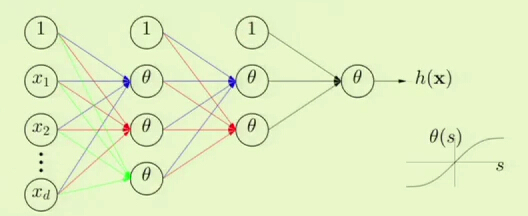其中第一列是输入数据，最右边的是输出，剩下的是隐藏层，该网络的层次 L = 3.

每条线表示一个权值，需要学习确定，而每个 θ 表示一个模型（任何模型，可以互不相等），每条线所属的层次跟其所指向的模型所在的层次相同.

下一层模型的输入是上一层模型的输出乘上对应的权值（所在的线的值）。
为了方便对神经网路进行讨论，这里假定每个模型的 θ(s) = (1-e^s)/(1+e^s), 处理的数据是二分类。

此外，定义如下符号：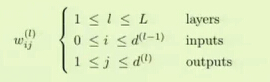w是我们需要学习的参数，对应图中的线，上标 l 表示当前的 w 属于第 l 层，下标表示该线出发的节点位置。j 表示该线指向的节点位置。值得注意的是 i 的取值范围，之所以能够去得到 i 是因为存在常数项： x0

于是我们有：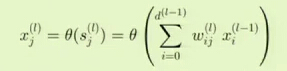其中 x 表示输出，s 表示输入。

当 l = 0 的时候表示的是原始的数据，这时候 0 < j < d + 1

对 x 的求解是一个递归的过程，先求出第 1 层，再求第二层...直到最终输出。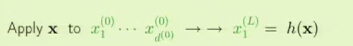该模型要解决如下两大难题：

1、generalization：众多的模型、参数、权重等导致自由度很大，正如在误差偏差分析里面所讲到的，假设集大会增大找到最优函数的难度。

2、optimization：应该如何进行学习才能把这么多参数学好？

第三部分将设法解决上述问题。
3、Backpropagation Algorithm ( 反向传播算法 )

这一部分基本是数学分析，看得有点晕，理解的也不是很好，只能是不断地截图了...

利用随机梯度下降法，每次只关注一个点。因此我们需要找出 Ein(W) 关于每一个数据的偏微分：▽e(W)。其中 e(W) = (h(xn),yn)。方便起见，令 e(W) = ( h(xn) - yn)^2（理论上可以用一切有效的误差度量函数）。

有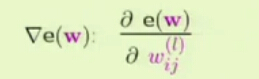=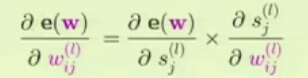因为 Sj(l) = Xi(l-1) * Wij(l) 所以(注：这里的 (l) 表示的是上标为 l )：令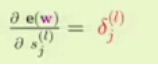，当 l = 1 的时候 Xi(l) 表示的是原始输入的数据，因此我们可以递归的求得上述等式右边的第二项，只要我们能够求出第一项就可以对 w 进行学习了。

为了求出第一项，先从最后一层开始，因为最后一层距离整个算法的输出最接近，且  θ(S1(L)) 就是 X1(L)，( 注：其中 L 是上标 )，有：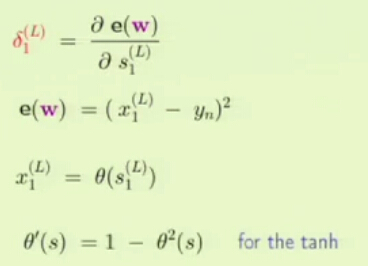当最后一层求出后，便可以递归的求出前面的值了。如下：( 之所以要求和是因为位于 l-1 层的节点受到 l 层的所有节点的反馈)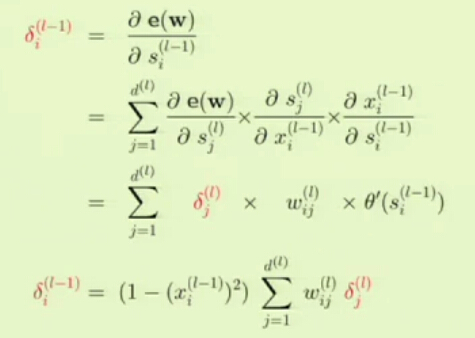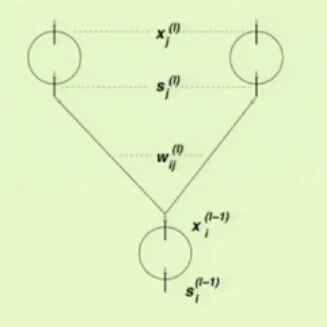下面是伪代码：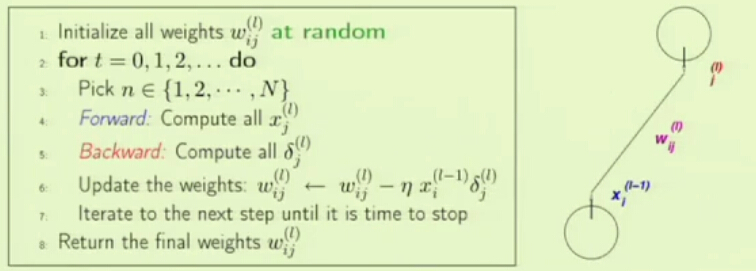总结：

第一部分承接了上一节课，介绍了一种更好的梯度测量方法，也为神经网络参数学习打下了基础。第二部分主要是介绍神经网络的基本概念及作用。最后一部分是基于数学的分析，从而把神经网络模型转换到计算机可以处理的层面，因此最后一部分是理论联系实际。

展开全文• I . 梯度下降 Gradient Descent 简介 ( 梯度下降过程 | 梯度下降方向 ) II . 梯度下降 示例说明 ( 单个参数 ) III . 梯度下降 示例说明 ( ...IV ....V ....VI . 批量梯度下降 VII . 随机梯度下降 VIII . 小批量梯度下降
文章目录I . 梯度下降 Gradient Descent 简介 ( 梯度下降过程 | 梯度下降方向 )II . 梯度下降 示例说明 ( 单个参数 )III . 梯度下降 示例说明 ( 多个参数 )IV . 梯度下降 总结 ( 定义损失函数 | 损失函数求导 )V . 梯度下降 方法VI . 批量梯度下降法VII . 随机梯度下降法VIII . 小批量梯度下降法

I . 梯度下降 Gradient Descent 简介 ( 梯度下降过程 | 梯度下降方向 )

1 . 后向传播算法 : 针对每个数据样本 , 从输入层到输出层传播输入 , 这是向前传播输入 , 然后从输出层向输入层传播误差 , 这是向后传播误差 ;

② 权重和偏置更新 : 传播误差过程中 , 对神经元网络中 , 单元连接的权重 , 和单元本身的偏置 , 进行更新 ;
③ 单元连接权重增量 : $\Delta w_{ij} = (l) Err_j O_i$
③ 单元偏置增量 : $\Delta \theta_j = (l) Err_j$

2 . 权重和偏置更新要求 : 更新的时候 , 涉及到 权重 和 偏置的增量 , 分别是 $\Delta w_{ij}$ 和 $\Delta \theta_j$ , 这两个增量值要使损失函数取最小值 , 并且该最小值越来越小 ;

3 . 权重和偏置更新方向 : 这两个增量的方向要求是 , 损失函数不仅要下降 , 并且损失函数下降的速度越快越好 , 这个损失函数下降最快的方向 , 就是梯度的反方向 , 梯度通常是对损失函数进行求导得到的 ;

损失函数 下降最快的方向 , 是梯度的反方向 ;
梯度通常是对损失函数进行求导得来的 ;
在某一点求导 , 就是这一点的曲线的切线的方向 ;
这里的方向只有两个 , 坐标轴正向 ( 从左到右 | 从负数到正数 | 增加 ) , 坐标轴反向 ( 从右到左 | 从负数到正数 | 减小 ) ;

4 . 权重和偏置更新步长 : 有了梯度以后 , 对 单元连接权重 和 单元偏置 参数进行更新时 , 还涉及到学习率 $l$ , 这个学习率 $l$ 又叫做超参数 ;

II . 梯度下降 示例说明 ( 单个参数 )

1 . 坐标轴说明 : 下图是损失函数 $f(\theta)$ , 横轴 $\theta$ 是指需要更新的 权重 或 偏置 参数 , 纵轴是损失函数 $f(\theta)$ 的计算结果 ;

2 . 损失函数最小值 : 取一个合适的 $\theta$ 值 , 使损失函数值 $f(\theta)$ 大小最小 , 此时是图中黄色的点对应的 $\hat{\theta}$ 值 ;

3 . 初始值 : 图中最左侧的值 , 标注为 Radom initial value , 即 随机初始值 ;

4 . 初始值变化趋势 : 每次对初始值进行一次变化 , 变化的方向是逐步使损失函数趋于最小 , 就是图中初始值开始到最小值的箭头方向 ;

5 . 梯度下降算法 : 梯度下降算法的目的是为了使损失函数 $f(\theta)$ 达到最小值 ;

6 . 梯度本质 : 梯度 或 求导 , 本质上是求某一点在曲线上的切线 , 切线的斜率表示的就是导数 ;

7 . 初始值累加方向 : 当 $\theta$ 是初始值时 , 切线的斜率是负数 , $\theta$ 是向右走 , 每次累加的 $\Delta\theta$ 是正数 , 因此 $\theta$ 值需要减去梯度的值 , 相当于加上了一个正数 , 因此说 $\theta$ 的方向与斜率是反方向 ( 方向指的是符号的正负号方向 ) , 即与梯度是反向方的 ; 每次累加的 $\Delta\theta$ 就是在 $x$ 轴上的两点之间的距离 ;

8 . 方向说明 ( 重点 ) :

损失函数 下降最快的方向 , 是梯度的反方向 ;
梯度通常是对损失函数进行求导得来的 ;
在某一点求导 , 就是这一点的曲线的切线的方向 ;
这里的方向只有两个 , 坐标轴正向 ( 从左到右 | 从负数到正数 | 增加 ) , 坐标轴反向 ( 从右到左 | 从负数到正数 | 减小 ) ;

9 . 学习步长 : 由初始值开始迭代 , 对 $\theta$ 参数进行更新 , 最终取得使损失函数值最小 , 即横轴坐标轴参数等于 $\hat{\theta}$ ; 该步长代表了该操作的效率 , 步长越大 , 速度越快 ;

10 . 梯度下降算法本质 : 对于当前的参数 $\theta$ 值 , 计算 $f(\theta)$ 的梯度 , 即导数 / 斜率 ( 负的 ) , 在梯度的反方向 ( 正数方向 ) 走一个步长 , 然后继续向前传播输入 , 再次向后传播误差时 , 继续计算其 $\theta$ 值对应的梯度值 , 迭代 $N$ 多次 , 即可得到使损失函数最小的参数值  ;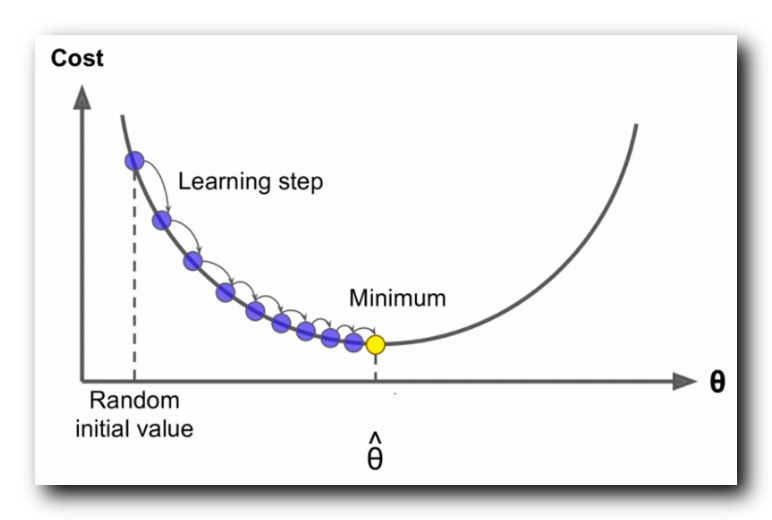上图是一个凸函数 , 最小值只有一个 , 比较好分析 , 实际情况是 , 同时存在多个甚至无数个能使 损失函数 $f(\theta)$ 取到最小值的 $\theta$ 值 ;

III . 梯度下降 示例说明 ( 多个参数 )

1 . 两个参数的损失函数 : 下图是有两个参数的情况下的损失函数情况 ;

2 . 损失函数示例 :
$z = x^2 + 2y^2$

① 分析基础 : 该损失函数 $z$ 有两个参数 , 该函数就不能再二维坐标轴中进行分析了 , 需要在三维坐标空间中进行分析 ;
② 坐标轴说明 : $z$ 轴是损失函数值 , $x$ 轴是 $x$ 参数的取值 , $y$ 轴是 $y$ 参数的取值 ;
③ 梯度下降要求 : 不仅要在 $x$ 轴损失函数下降最快 , 在 $y$ 轴损失函数也要下降最快 ; 如下图中的蓝色箭头方向 ;

3 . 参数说明 :

如果有 $1$ 个参数 , 就是在二维空间中进行分析 , 如在 $x$ 轴 和 $y$ 轴平面上分析 ;
如果有 $2$ 个参数 , 就是在 $3$ 维空间中进行分析 , 如在 $x$  , $y$ 轴 和 $z$ 轴 三维空间上分析 ;
$\vdots$
如果有 $n$ 个参数 , 就是在 $n + 1$ 维空间中进行分析 ;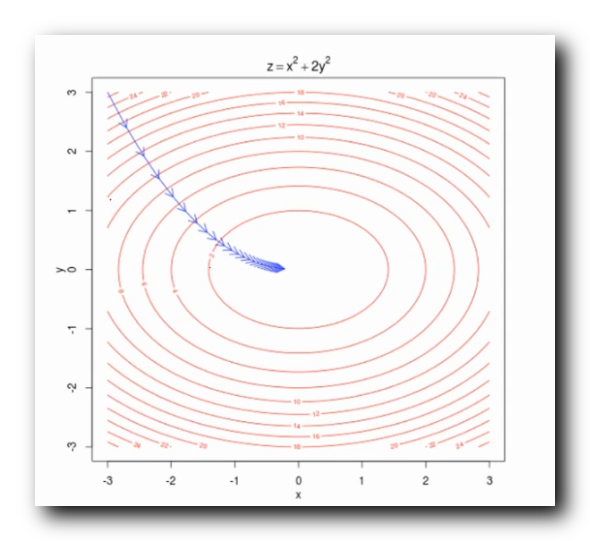最终的效果可能不是这样的 , 实际可能会存在很多弯曲波折 , $x , y$ 参数数值 , 在各轴上 , 按照锯齿形状下降 , 但是大概的趋势是这样的 ;

IV . 梯度下降 总结 ( 定义损失函数 | 损失函数求导 )

1 . 单个参数的梯度下降算法公式表示 :

$\theta^{new}_j = \theta^{old}_j - \alpha \frac{ \partial }{ \partial \theta^{old}_j} J (\theta)$

$\theta_j$ 表示第 $j$ 个参数 ;
$\theta^{new}_j$ 表示新的第 $j$ 个参数 ;
$\theta^{old}_j$ 表示旧的第 $j$ 个参数 ;
$\alpha$ 指的是学习率 , 或梯度下降的步长 ;

如果是单个参数值 , 是对 目标函数 / 损失函数 $J (\theta)$ 关于当前的第 $j$ 个参数 $\theta_j$ 进行求导 , 只对一个参数进行求导 ;

2 . 使用矩阵的形式表示多个参数的梯度下降算法 :

$\theta^{new} = \theta^{old} = \alpha \nabla_\theta J (\theta)$

$\theta$ 表示很多参数的矩阵 ;
$\theta^{new}$ 表示新的参数的矩阵 ;
$\theta^{old}$ 表示旧的参数矩阵 ;
$\alpha$ 指的是学习率 , 或梯度下降的步长 ;

$\nabla_\theta J (\theta)$ 表示梯度的反向方 , 这是对 目标函数 / 损失函数 $J (\theta)$ 关于 $\theta$ 进行求导 , 注意 $\theta$ 是很多参数组成的矩阵 , 需要对每个参数进行求导 , 即偏导 , 然后将这些偏导整合起来 ;

3 . 梯度下降算法实现 :

① 定义损失函数 : 先定义损失函数 , 一般是误差平方和 ;
② 求参数增量 : 对损失函数进行求导 , 使用导数 乘以 学习率 ( 步长 ) , 得到一个 参数增量 , 这里的参数指的是 权值 和 偏置 ;
③ 伪代码实现 :
//迭代循环执行下面的代码 , 每循环一次 , 梯度下降一次 , 损失函数的值就会变小一次
while true :
//对损失函数进行求导 , 也就是评估梯度 , J 代表损失函数 , theta 代表参数值
//	本质是 对损失函数 J 关于 theta 求导
theta_gradient = evaluate_gradient ( J , corpus , theta )

//左侧的 theta 是新的 theta , 右侧的 theta 是旧的 theta
//	alpha 是学习率 , 也是梯度的步长 , theta_gradient 是损失函数导数
theta = theta - alpha * theta_gradient


V . 梯度下降 方法

1 . 常用的梯度下降方法 :

① 批量梯度下降法 : Batch Gradient Descent ;
② 随机梯度下降法 : Stochastic Gradient Descent ;
③ 小批量梯度下降法 : Mini-batch Gradient Descent ; 介于上述两者之间 ;

VI . 批量梯度下降法

批量梯度下降法 : 梯度下降的最常用方法 , 反向传播误差时 , 使用误差更新参数时 , 参考所有样本的误差更新 权值 和 偏置参数 , 如果有 $n$ 个样本 , 每次迭代时 , 将这 $n$ 个样本全部处理一遍 , 每次迭代都要使用所有的样本进行参数更新 ; 公式如下 :

$\theta' = \theta - \alpha \sum_{j=1}^n( h_\theta ( x_0^{(j)}, x_1^{(j)}, \cdots , x_n^{(j)} ) - y_j) x_i^{(j)}$

样本个数 : $n$ 代表样本个数 ;
梯度计算 : 此处计算梯度 , 使用了所有的样本的梯度数据 ;

VII . 随机梯度下降法

随机梯度下降法 : 求梯度的时候 , 随机选择一个样本进行 , 使用该样本的误差更新参数 ; 公式如下 , 相对于批量梯度下降法的公式只是删除了 总和 符号 , 不再累加所有的样本误差数据 ;

$\theta' = \theta - \alpha ( h_\theta ( x_0^{(j)}, x_1^{(j)}, \cdots , x_n^{(j)} ) - y_j) x_i^{(j)}$

VIII . 小批量梯度下降法

小批量梯度下降法 :

① 方法引入 : 上述的批量梯度下降法 , 使用所有的样本 , 训练时间很长 , 但是预测准确度很高 ; 随机梯度下降法 , 训练速度很快 , 准确度无法保证 ; 这里引入一种介于上述两个方法之间的一种方法 , 即小批量梯度下降方法 ;
② 参数更新方式 : 数据集有 $n$ 个样本 , 采用其中的 $m$ 个样本的子数据集 进行迭代更新参数 ;
③ 公式 :
$\theta' = \theta - \alpha \sum_{j=1}^m( h_\theta ( x_0^{(j)}, x_1^{(j)}, \cdots , x_n^{(j)} ) - y_j) x_i^{(j)}$
注意上述 求和的是 子数据集的 $1$ 到 $m$ 索引 ;


展开全文后向传播算法 梯度下降 损失函数
• 通过介绍评定模型，随机梯度下降，生物启示和感知器系统，讲师用视图和数学解析式具体地解说了神经网络的执行过程以及原理. 课程大纲： 1、Stochastic Gradient Descent ( 随机梯度下降 ) 2、Neural Network ...


课程简单介绍：
本节课主要介绍人工神经网络.通过介绍评定模型，随机梯度下降法，生物启示和感知器系统，讲师用视图和数学解析式具体地解说了神经网络的执行过程以及原理.
课程大纲：
1、Stochastic Gradient Descent ( 随机梯度下降法 )
2、Neural Network Model ( 神经网络模型 )
3、Backpropagation Algorithm ( 反向传播算法 )
4、Summarize
1、Stochastic Gradient Descent
在上一节课里，我们利用梯度下降法找到最优的方向，然后向该方向移动一小步，可是很有可能会导致陷入一个差的局部最优解，并且每次移动都要计算所有的点，因此计算量大。那假设每次移动仅仅看一个点，依据当前的点找到最优的方向进行移动，当所有的点都被选择后，理论上总的移动方向是跟之前的方法一致的，由于数学期望是相等的。可是利用随机的选取一个点，却有可能让我们逃脱很差的局部最长处的陷阱，当然无法逃脱所有的陷阱。那是由于当你随便选取一点的时候，它的方向并不一定就是最优化的那个方向，因此下一步有可能移动到高点去，然后在新的位置又一次寻找出路，这样就有更大的可能性获得较好的局部最长处。考虑以下的图片，在左图中，当我们第一次选择的初始化位置是第一个黑点的时候，那么依据总体梯度下降法，就会陷入那个很糟糕的局部嘴有点去了。然而假设我们选择的是随机梯度下降法，就有可能由于某个点的方向是偏离最长处的而使得前进的方向是想着右边的，这样就能够跳过该陷阱了。由图中，依据总体梯度下降法，当遇到平缓区域的时候，该方法就会觉得找到最长处了，就会停滞不前。而随机梯度下降法却由于不同点的方向不一致从而推动了算法继续向前移动，于是我们再次逃脱了该陷阱了。应用随机梯度下降法有例如以下优点：
1、计算代价小，由于每次仅仅选取一个点进行计算
2、随机性有助于逃脱局部最小点，找到较好的最小点
3、简单，由于每次仅仅选取一个点进行计算
2、Neural Network Model ( 神经网络模型 )
在学习感知器模型的时候我们知道当输入数据点超过三个的时候，最坏情况下我们没办法把所有的点所有正确分类，即 dVC = 3。然而假设利用两个感知器模型进行分类，然后再综合这两个模型的结果得到最总的结果，我们就有办法把所有的 4 个点所有分类，例如以下图：对于很多其它的点，我们能够利用很多其它的模型进行分类。通过综合不同模型的结果，就有可能通过感知器模型找到最优的解（权重）。
利用下面图形进行直观的解释：上图中共同拥有第一列是输入，后面三列表示三层模型，共 5 个感知器模型（每一个圆圈表示一个感知器模型，也叫一个神经元或节点）。最后的感知器输出的就是终于的结果。
每一条线上都有一些数值，这些便是我们须要学习的权重（參数），開始的时候是未知的。上述模型事实上就是一个神经网络。
理论上通过这些感知器的排列组合(随意个)，能够产生不论什么输出。
定义：
神经网络的模型是通过组合不同的简单模型而得到的一个综合的模型，组合方式例如以下图所看到的：当中第一列是输入数据，最右边的是输出，剩下的是隐藏层，该网络的层次 L = 3.
每条线表示一个权值，须要学习确定，而每一个 θ 表示一个模型（不论什么模型，能够互不相等），每条线所属的层次跟其所指向的模型所在的层次同样.
下一层模型的输入是上一层模型的输出乘上相应的权值（所在的线的值）。
为了方便对神经网路进行讨论，这里假定每一个模型的 θ(s) = (1-e^s)/(1+e^s), 处理的数据是二分类。
此外，定义例如以下符号：w是我们须要学习的參数，相应图中的线，上标 l 表示当前的 w 属于第 l 层，下标表示该线出发的节点位置。j 表示该线指向的节点位置。值得注意的是 i 的取值范围，之所以可以去得到 i 是由于存在常数项： x0
于是我们有：当中 x 表示输出，s 表示输入。
当 l = 0 的时候表示的是原始的数据，这时候 0 < j < d + 1
对 x 的求解是一个递归的过程，先求出第 1 层，再求第二层...直到终于输出。该模型要解决例如以下两大难题：
1、generalization：众多的模型、參数、权重等导致自由度非常大，正如在误差偏差分析里面所讲到的，如果集大会增大找到最优函数的难度。
2、optimization：应该怎样进行学习才干把这么多參数学好？
第三部分将设法解决上述问题。
3、Backpropagation Algorithm ( 反向传播算法 )
这一部分基本是数学分析，看得有点晕，理解的也不是非常好，仅仅能是不断地截图了...
利用随机梯度下降法，每次仅仅关注一个点。因此我们须要找出 Ein(W) 关于每个数据的偏微分：▽e(W)。当中 e(W) = (h(xn),yn)。方便起见，令 e(W) = ( h(xn) - yn)^2（理论上能够用一切有效的误差度量函数）。
有=由于 Sj(l) = Xi(l-1) * Wij(l) 所以(注：这里的 (l) 表示的是上标为 l )：令，当 l = 1 的时候 Xi(l) 表示的是原始输入的数据，因此我们能够递归的求得上述等式右边的第二项，仅仅要我们能够求出第一项就能够对 w 进行学习了。
为了求出第一项，先从最后一层開始，由于最后一层距离整个算法的输出最接近，且  θ(S1(L)) 就是 X1(L)，( 注：当中 L 是上标 )，有：当最后一层求出后，便能够递归的求出前面的值了。例如以下：( 之所以要求和是由于位于 l-1 层的节点受到 l 层的全部节点的反馈)以下是伪代码：总结：
第一部分承接了上一节课，介绍了一种更好的梯度測量方法，也为神经网络參数学习打下了基础。第二部分主要是介绍神经网络的基本概念及作用。最后一部分是基于数学的分析，从而把神经网络模型转换到计算机能够处理的层面，因此最后一部分是理论联系实际。


展开全文• 【20200506】数据挖掘DM课程课业打卡九之人工神经网络 Artificial Neural Networks -ANN 一、课业打卡八之支持向量机 填空题 二、知识点巩固 1、关于人工神经网络 2、人工神经网络——感知器 3、多层人工神经网络 4...
• 神经网络：巨量并行，智慧无限 神经元（感知机，Perceptrons），注意常数项w0,不然总是经过原点 如何设置和调整感知机输入的权重？ 梯度下降，知错就改（Stochastic Learning） 如何解决线性不可分问题？ 线性...深度学习 机器学习 人工智能
• 数据处理分组(GMDH)的多层算法(MIA)是通过利用遗传算法的选择程序来实现的，该算法包含了为获取更少误差而生成的最优神经元的克隆操作．选择程序根据适应度以及网络输入的某种概率从已有的神经元中为新神经元寻找...
• 决策树： 贝叶斯定理： ...神经网络： 梯度下降： BP算法 网络例子：Online Demo http://neuron.eng.wayne.edu/software.html http://facstaff.cbu.edu/~pong/ai/hopfield/hopfieldap...
• 本文是对《Python数据分析与挖掘实战》实战篇第一部分——电力窃漏电用户自动识别中上机实验的一个记录。 实验分为两个部分： 利用拉格朗日插值进行缺失值的补充 构建分类模型对窃漏电用户进行识别 第一部分...python 应用
• 常用的分类算法包括：决策树分类法，朴素的贝叶斯分类算法(native Bayesian classifier)、基于支持向量机(SVM)的分类器，神经网络法，k-最近邻法(k-nearest neighbor，kNN)，模糊分类法等等 决策树分类法 机器...
• ## 图像数据挖掘

千次阅读 2011-08-07 15:28:56
数据挖掘是在大量数据中发现隐含其中的模式、特征、规律和知识。根据实际的模式可以分为分类、聚类、回归、...常规分类有：贝叶斯、决策树、最大似然发、最小距离神经网络、支持向量机、模糊分类、k-均值，如果在数据库
• 答：空间数据挖掘的常用方法有：统计，聚类方法，关联规则发掘方法，Rough集方法，神经网络方法，云理论，证据理论，模糊集理论，遗传算法等算法（出自丁信宙，仇环，苏晓庆. 基于云理论的缺损数据推理和预测 山东...
• 数据挖掘课程刚刚讲完人工神经网络ANN然后介绍了一些相关的概念 为了训练得到神经网络参数w b 而使用的梯度下降啊 反向传播啊 看着ppt 听着老师讲课 然后就… 没听懂 咳咳 所以 逛逛CSDN巩固下神经网络基础 学习...感知机 深度学习 误差逆传播算法
• 数据挖掘涉及的学科领域和方法很多,有多种分类。根据挖掘任务分,可分为分类或预测模型发现、数据总结、聚类、关联规则发现、序列模式发现、依赖关系或依赖模型发现、异常和趋势发现等等;根据挖掘对象分,有关系...数据结构 算法 领域模型
• 除了标准最小二乘法、逐步和Logistic等传统的回归建模分析之外，JMP还提供了包括决策树、神经网络、时间序列、随机森林、提升树、朴素贝叶斯等众多建模技术。 在数据挖掘方面，JMP既提供了探索性数据挖掘方法，...数据分析 大数据 机器学习
• 本文检索了近年来基于数据挖掘的会计舞弊识别方面的文献,归类比较挖掘数据、算法以及分类器评价方法和分类效果,为投资者、监管部门和审计师在舞弊识别中选用适当数据和挖掘技术及优化模型提供借鉴。现有研究多对舞弊...
• 答：空间数据挖掘的常用方法有：统计，聚类方法，关联规则发掘方法，Rough集方法，神经网络方法，云理论，证据理论，模糊集理论，遗传算法等算法（出自丁信宙，仇环，苏晓庆. 基于云理论的缺损数据推理和预测 山东...分布式计算 机器学习
• 文中首先分别给出了人工智能与数据挖掘的发展简史以及主要的研究领域和研究途径,而后给出了三个典型算例,分别对矿井作业的安全问题采用小波神经网络方法进行评价,对某系统的性能用模糊神经网络进行预测,对飞行员的...
• 作者：杨晓辉 天津工程师范学院计算机系 关键词：生态安全；数据挖掘神经网络；关联规则；梯度
• 向AI转型的程序员都关注了这个号???大数据挖掘DT数据分析 公众号： datadw最近在看深度学习的东西，一开始看的吴恩达的UFLDL教程，有中文版...反向传播其实是神经网络的基础了，但是很多人在学的时候总是会遇到一些问
• 上一周去听了宫老师的K-NN，及神经网络的算法课程。这一周本来准备深入研究一下，回来后，看到同事桌上的魔方，就开始还原了起来。实际上，从魔方的角度来思考我们数据挖掘里的一些算法，发现，另有一番收获。 先说...
• ## 数据挖掘分类算法

千次阅读 2018-04-02 15:17:08
转载自：https://www.cnblogs.com/heiye123/articles/7440687.html讨论QQ：465925664目录 1.线性判别 2.... 人工神经网络(ANN=Artificial Neural Networks) 7.支持向量机 SVM 正文常见分类模型与...
• BSYES算法 论文+答辩PPT 目录 ...3．2方法论（神经网络分类） 7 3．3软件平台（MATLAB） 7 第四章 8 4．1 我的工作 8 4．2 工作结果小结 8 第五章 总结与展望 8 第六章 致谢 8 第七章 参考文献 8